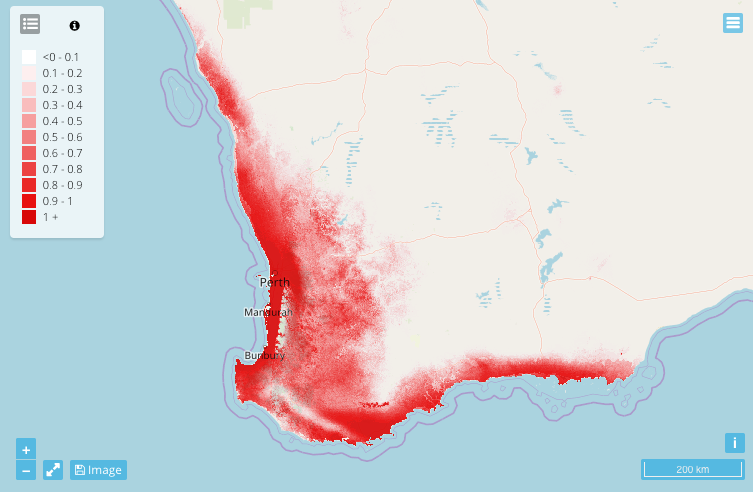Why conduct an ensemble analysis?

Instead of using a single-model, or single-emissions-scenario approach to investigating species distributions you can conduct an Ensemble Analysis experiment. An ensemble analysis reduces the uncertainty of a single model or emission scenario by combining the results of multiple. An ensemble analysis experiment provides visual outputs based on statistics including the mean, minimum, maximum, variance and percentiles across multiple algorithms or climate scenarios. For example, you can synthesise the results of two or more related but different analytical models, such as multiple algorithms in an SDM, or combine the results of two or more related but different climate models/scenarios from a Climate Change experiment.

To do this you must first conduct a species distribution model (SDMor Climate Change experiment (CCin EcoCommons and then conduct an Ensemble Analysis experiment. Once you have run an experiment in EcoCommons, the results page shows you a variety of different outputs. Below is an explanation on how to interpret the outputs of an Ensemble analysis experiment.

Summary mean

The ensemble analysis experiment produces multiple distribution maps. The first is the summary mean which shows the average predicted distribution of your species across multiple algorithms and baseline conditions from the SDM or climate scenarios from the CC Experiment. The prediction is visualised as the suitability of a grid cell on a scale from 0 to 1, where 0 refers to very low suitability and 1 refers to very high suitability.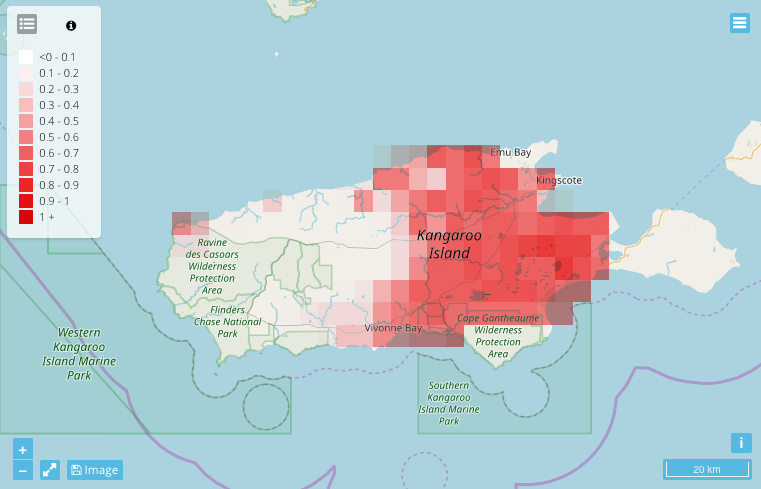Summary minimum and maximum

The summary minimum map shows the minimum predicted distribution of your species across multiple algorithms and baseline conditions from the SDM or climate scenarios from the CC Experiment. The summary maximum map shows maximum predicted distribution. The prediction is visualised as the suitability of a grid cell on a scale from 0 to 1, where 0 refers to very low suitability and 1 refers to very high suitability.

Minimum                                                                    MaximumSummary variance

The summary variance map shows the potential variance within the prediction distribution. The variance is visualised as the suitability of a grid cell on a scale from 0 to 1, where 0 refers to very low variance and 1 refers to very high variance.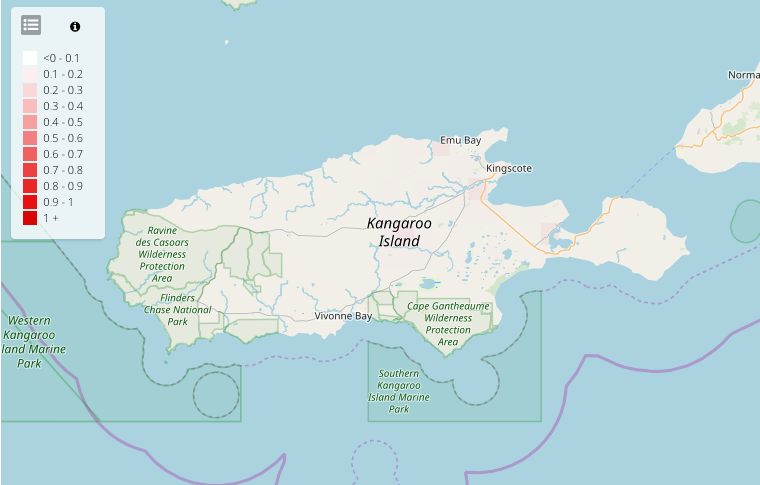Percentiles

The remaining output maps show the predicted distribution within different percentiles of the distribution. These include the 5th, 10th, 50th, 90th and 95th percentiles. The prediction is visualised for each percentile as the suitability of a grid cell on a scale from 0 to 1, where 0 refers to very low suitability and 1 refers to very high suitability.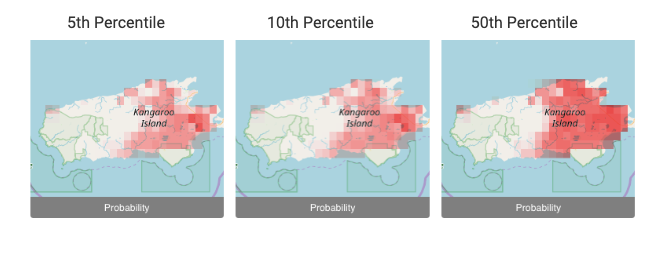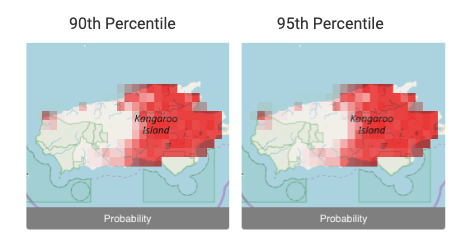Change in probability map

If you conducted your ensemble analysis based on a CC experiment there will be additional output. The Change in probability map shows the difference in the predicted probability between SDM probability and the CC experiment probability across the algorithms and climate scenarios. This prediction is visualised as the probability of occurrence for any location between current and future projections. The map shows a grid cell on a scale from -1 to 1, where -1 refers to a low predicted probability and 1 refers to a high probability.Change in species range map

The Change in species range map is generated based on binary model outputs from the SDM and CC experiments. The predicted probability maps from the SDM and CC are transformed from a continuous probability map (with values ranging from 0 to 1) to a binary map (presence/absence) using the threshold selected on the Source Experiment tab when designing the CC experiment.

The resulting change in species range map indicates for each grid cell across multiple climate scenarios whether:

Blue: the species is present in both SDM and CC predictions, thus no change.

Orange: the species is present in SDM prediction, but absent in CC prediction, thus decrease in range.

Green: the species is absent in SDM prediction, but present in CC prediction, thus increase in range.

White (no colour): the species is absent in both SDM and CC predictions, thus no change.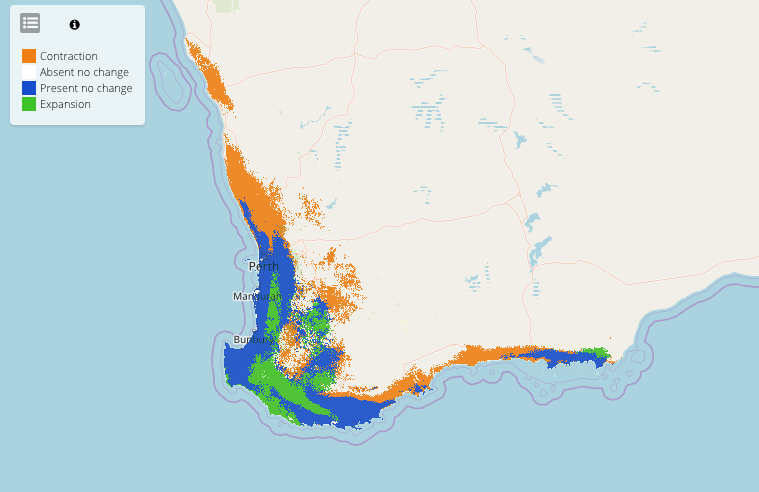Change in Species Range Table

The change in species range table shows the number and percentage of grid cells for each of the categories in the species range change map + area km2 calculated based on actual size of grid cell. This table also shows the range change in km2 for example this table shows the suitable habitat will contract 36760.487 km2 and expand 16642.414 km under the future climate scenarios in the ensemble analysis.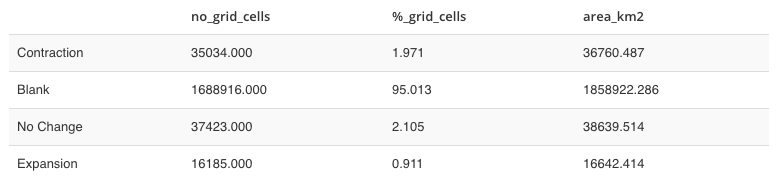SDM Mean

The SDM mean map shows the original SDM predicted distribution map. This allows for an easy comparison between the original results and the ensemble results. The prediction is visualised as the suitability of a grid cell on a scale from 0 to 1, where 0 refers to very low suitability and 1 refers to very high suitability.